# Asymmetric Cryptography: RSA (II)

In the previous post we talked about RSA and how its maths mork. As I promised, today I bring you a real implementation of the algorithm, using Python. The scope is to understand how RSA works in order to use it in a secure way.

#### In previous posts…

RSA’s maths are not really complex. However, they need a lot of variables, so it can become a great headache to remember them all. Here I show the variable we are using and their names:

• p and q: initial random integers (they should be large integers)
• n: the modulus we use to encrypt and decrypt.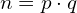.
•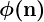: Euler’s Phi Function. It show the amount of relative primes to n. Since p and q are relative primes,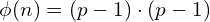. This is the modulus we use to calculate the keys.
• e: public key integer. We usually take 65537. As a remainder, the real public key is the pair (e, n).
• d: private key. It is the inverse of e modulo n.
• m: message we want to encrypt and send.
• c: encrypted message.

#### Environment

We want to understand how RSA works, so we are explicitly avoiding pre-implemented libraries. You can always use OpenSSL or similar tools to encrypt and decrypt messages. The code I will show you is written in Python 2.7, so you can use any interpreter to run it.

However, what I prefer is to use Jupyter Notebook. More precisely, I recommend SageMath: a software designed for mathematics purposes, which can run over a Jupyter Notebook. This is the easiest way to follow today’s post. When playing CTFs, a lot of cryptographic challenges can be solved using this environment, since SageMath is able to run optimized Number Theory and Algebra functions.

#### Hands on

We want to write a RSA implementation. We can do it roughly or carefully. What I’m trying to say is that it is very, very easy to make a mistake when writing your own Cryptography.

Our implementation should meet the following requirements:

1. Use a secure RNG (Random Number Generator).
2. Use reasonable secure lengths.
3. Avoid insecure number combinations.
4. NEVER repeating important information (like the modulus).

At the end of the post you will find the full code. In the next sections we will show fragments of this code to explain its content.

#### Obtaining Random Numbers

A RNG is an algorithm to generate pseudo-random number. What does it mean?

Computationally speaking, it does not exist such thing as randomness. Computers are deterministic machines, so the only way we have to obtain “random” numbers using a computer lies in using deterministic sources to simulate randomness.

As an example, we could use the system timestamp, consisting in hours, minutes and seconds. What is the probability for the seconds to have a value of ‘1’? We could think it is 1/60, as we could think that this probability is the same for every value between 0 and 59.

However, this is a really simple example, which would be insecure when creating a real RNG. There is a whole theory built around RNG, and this is not the scope of the post.

There are several libraries and functions to obtain secure random numbers. An example is pycryptodome, which is the library we have chosen.

In the first place, we are going to obtain two pseudo-random numbers, p and q. We will use the getPrime() function for that. Its second argument is the Random function we want to use, which is get_random_bytes(), considered a “secure” Python function.

Nowadays, the recommended length for RSA keys is 1024 bits, so the numbers p and q should be about 512 bits. Besides,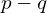should not be small!

We can now obtain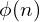, which will be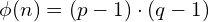(remember the previous post?).

p = Crypto.Util.number.getPrime(512,randfunc=Crypto.Random.get_random_bytes)
q = Crypto.Util.number.getPrime(512,randfunc=Crypto.Random.get_random_bytes)
n = p * q
PHI = (p-1)*(q-1)

#### Key Selection

The next step is to generate the public and private keys. For the public one, we have two options: we can take a random e (be sure that e is relatively prime to) or we can take the number 65537, which is a proven secure RSA number.

We will take 65537 for simplicity.

Private key is determined by the public one. We take the inverse of e modulo. For this purpose, we can use inverse(a,b) function, which calculates the inverse of a modulo b.

e = 65537
d = inverse(e, PHI)

#### Encoding

At this moment you may be asking How are is RSA supposed to work with letters instead of numbers?

One of the most common techinques is taking the hexadecimal representation of the string and casting it into bytes, meaning we will take the binary representation of our message and read it as a base-2 integer.

encoded = int.from_bytes(m.encode('utf-8'), byteorder='big')

Note that we are taking the hexadecimal encoding of the message m, using m.encode(‘utf-8’). After that, we use int.from_bytes() to get the binary string and read it as a big-endian integer.

The decoding process is similar:

hex = ('%x' % encoded)
bytes.fromhex(hex).decode('utf-8')

What we are doing here is to cast the integer to its hexadecimal notation, in order to decode it into a UTF-8 string.

Once we encrypted the message, however, its better to leave it in its hexadecimal notation, since there will be characters which are not included in the UTF-8 charset.

Another way to do it is to take its Base64 representation.

An example of RSA encrypted message, in hexadecimal notation, would be:

104353aae6eb1c9b71bf4fee598016fb9c20607eb4920662b6924e76f16cc049375066ec7b69107bf2693ec724be8dd78e153b123d4a059fb6cfd95d0713c7feba7e6313351511c9aaf90ccecfd6375edae1335c13fe158c44883dd3f6a5fcfa953488aec1fd1800943c70bfcc5c15c6c0ec5e92cdd3f769dd6fd46fd3613717

At the end of the post, two functions have been defined to encode and decode strings: decode_as_hex() and encode_from_hex().

#### Encryption and Decryption

Once we have encoded the message, this is the easiest part.

As we explained in the previous post, when encrypting a message we need to calculate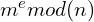. Decryption process is really similar: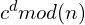. The pow(a,b,m) function will calculate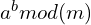, so it will get our job done.

# Let encoded be the integer-encoded message and c the encrypted message
c = pow(encoded, e, n)

# Decryption
decrypted = pow(c, d, n) # We need to decode the "decrypted" string to obtain the initial message

## PKCS#1 Standard

We have our public and private keys, and we are capable of encrypting and decrypting a message. However, we need a standardized way to share our keys and messages in order to facilitate a communication.

PKCS#1 was designed with this purpose. It is an widely spread international standard, used in tools and libraries like OpenSSL. It defines how public and private keys should be stored and shared. Actually, when we click on the SSL certificate of a webpage, we will see its public key/certificate in this format.

pyCryptodome includes functions to export our keys using this standard:

from Crypto.PublicKey import RSA
privateKey = RSA.construct((n, e, d))
publicKey = RSA.construct((n, e))

We we call publicKey.exportKey(), we will obtain:

-----BEGIN PUBLIC KEY-----MIGeMA0GCSqGSIb3DQEBAQUAA4GMADCBiAKBgH2LjI44vqO68tuNk/bxNYYd6FJa3R3i7HzgiyvxJnavoYy2GcMMmdPCVC2OqCj5a2wo4A4GqHCPTWh43h3CqP4njUHMs3LXVxw/CInpeKanYsaDqZhmn6AEjM1vgUk/cZQYRYze0MOb6MgGkxR3I4VoBwLH5dsYS6tM2iuarX1DAgMBAAE=-----END PUBLIC KEY-----

Which follows the PKCS#1 standard, with ASN.1 syntax.

#### The Code

from Crypto import *
from Crypto.Util import *
from Crypto.Util.number import *

# Obtaining keys

# Get random 512-digit prime numbers
p = Crypto.Util.number.getPrime(512,randfunc=Crypto.Random.get_random_bytes)
q = Crypto.Util.number.getPrime(512,randfunc=Crypto.Random.get_random_bytes)

n = p * q
PHI = (p-1)*(q-1)

# Public key integer
e = 65537

#Private key integer as modular inverse of e over PHI
d = Crypto.Util.number.inverse(e, PHI)

def encode(message):
return int.from_bytes(m.encode('utf-8'), byteorder='big')

def decode(encoded):
hex = ('%x' % encoded)
return bytes.fromhex(hex).decode('utf-8')

def decode_as_hex(encoded):
return ('%x' % encoded)

def encode_from_hex(hex):
return int(hex,16)

# Encrypted RSA messages will be shown in hex encoding
# because UTF-8 wont recognize some characters.
def encrypt(message, e, n):
encoded = encode(message)
c = pow(encoded, e, n) # encoded ^ e % n
return decode_as_hex(c)

# Input will be hex encrypted message
def decrypt(hex, d, n):
encoded = encode_from_hex(hex)
c = pow(encoded, d, n) # encoded ^ d % n
return decode(c)

m = "No one can read me muahahaha"
encrypted = encrypt(m, e, n)
print("Encrypted message with hex encoding:")
print(encrypted)
print("\nDecrypted message:")
print(decrypt(encrypted, d, n))